Home > CC2MN > Chapter cc18 > Lesson cc18.1.3 > Problem8-42

8-42.
1. Complete a portions web for each of the following fractions. Homework Help ✎

1.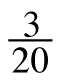2.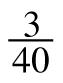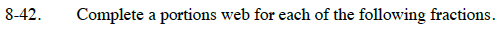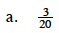Divide the numerator by the denominator to get a decimal number.

Multiply the decimal number by 100 to get a percent.

15%, 0.15, fifteen hundredths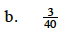Follow the steps you used in part (a).